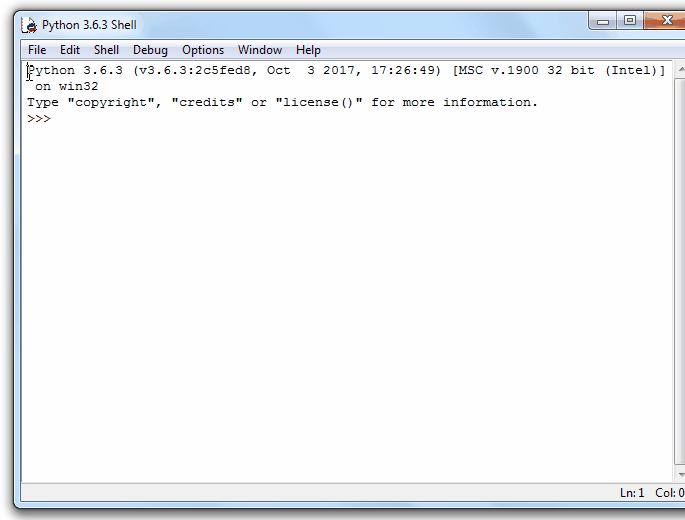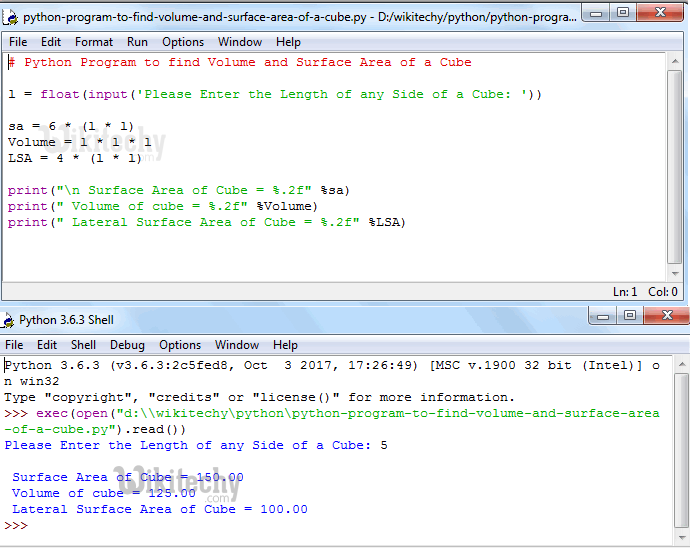# python tutorial - Python Program to find Volume and Surface Area of a Cube - learn python - python programming

• To write Python Program to find Volume and Surface Area of a Cube with example.
• Before we step into the program, Let see the definitions and formulas behind Surface area of a Cube and Volume of a CubeLearn Python - Python tutorial - Python Program to find Volume and Surface Area of a Cube - Python examples - Python programs

## CUBE

• All the edges in the Cube has same length. OR We can say, Cube is nothing but 6 equal squares.

## Surface Area of a Cube

• If we know the length of any edge in Cube then we can calculate the surface area of a Cube using the formula:
• Surface Area of a Cube = 6l² (Where l is the Length of any side of a Cube).
• Area of a square = l² Since the Cube is made of 6 equal squares, Surface Area of a Cube = 6l²
• If we already know the Surface Area of Cube and then we can calculate the length of any side by altering the above formula as:

## Volume of a Cube

• The amount of space inside the Cube is called as Volume. If we know the length of any edge of a Cube then we can calculate the Volume of Cube using formula:

## Python Program to find Volume and Surface Area of a Cube

• This program allows user to enter any side of a Cube.
• Using this value, this Python program will calculate the Surface Area of a cube, Volume of a cube and Lateral Surface Area of a Cube as per the formulas.

## Analysis

• Below statements will ask the user to enter the length value and it will assign the user input values to respected variable.
• Next, We are calculating Volume, Surface Area and Lateral Surface Area of a Cube using their respective Formulas:
• Following print statements will help us to print the Volume, Lateral Surface Area and Surface area of a Cube

## OutputLearn Python - Python tutorial - Python Program to find Volume and Surface Area of a Cube - Python examples - Python programs

• We entered the Length of any side of a Cube = 5

The Surface Area of a Cube is
Surface Area of a cube = 6 * length * length
Surface Area of a cube = 6 * 5 * 5
Surface Area of a cube = 150

The Volume of a Cube is
Volume of a cube = length * length * length
Volume of a cube = 5 * 5 * 5
Volume of a cube = 125

The Lateral Surface Area of a Cube is
Lateral Surface Area of a cube = 4 * length * length
Lateral Surface Area of a cube = 4 * 5 * 5
Lateral Surface Area of a cube = 100

• In the above example we got Surface area = 150 when the length = 5. Let us do the reverse approach (Calculate the length of a Cube using Surface Area = 150)

Length of a cube = √sa / 6
Length of a cube = √150 / 6
Length of a cube = √25
Length of a cube = 5

## Python Program to find Volume and Surface Area of a Cube using functions

• This program allows user to enter any side of a Cube.
• We will pass the value to the function argument and then it will calculate the Surface Area of a Cube, Lateral Surface Area of a Cube and Volume of a Cube as per the formula.

## OutputLearn Python - Python tutorial - Python Program to find Volume and Surface Area of a Cube using functions - Python examples - Python programs

## Analysis

• First, We defined the function with one argument using def keyword.
• It means, User will enter the length of a cube.
• Next, We are calculating the Surface Area of a Cube, Lateral Surface Area of a Cube and Volume of a Cube as per the formula

NOTE: We can call the function with arguments in .py file directly or else we can call it from the python shell. Please don’t forget the function arguments